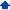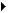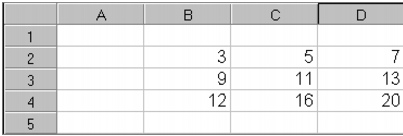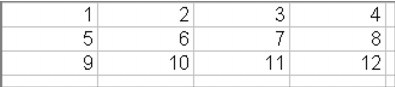### Reference: Working with formulas and functions

Rules of mathematics and syntax are applied when you enter or modify formulas and functions in spreadsheets. Using arguments, dates, and arrays in functions allow you to extend your calculations. As well, setting cell names and addresses simplifies entering formula and function expressions.When you create formulas that reference cells you can refer to the cells by their cell address. This address is the intersection of the column letter and the row number, such as A15 or B6. When referring to a cell on a different spreadsheet, first specify the name of the spreadsheet; for example, C:A15. You can also specify a block of cells in this way by creating a cell address range, such as A5..D16.

When you reference data in formulas, functions, or macros, you can refer to the cell by relative cell address, absolute cell address, or cell name.

When you set a relative cell address, the formula calculates the data from cells relative to its current location in the spreadsheet. When you change the address of a cell that contains a formula, the formula calculates the address relative to its new location. For example, if you create a formula in cell A3 to add cells A1 and A2, and then you move the formula to cell D3, the formula adjusts to add cells D1 and D2.

By default, cell formulas calculate relative cell addresses.

When you set an absolute cell address in a formula, the formula always references the same cells regardless of their location on the spreadsheet. For example, if you create a formula in cell A3 to add cells A1 and A2, and then you move the formula to cell D3 on the same spreadsheet, the formula still calculates cells A1 and A2.

You can set an absolute address by typing a dollar sign before the part of the cell address you want to make absolute. For example,

 • typing \$A\$1 makes both coordinates of address A1 absolute
 • typing \$A1 locks the address into column A, but lets the row coordinate change
 • typing A\$1 locks the address into row 1, but lets the column coordinate change
 • typing \$A:A\$1 locks the address into sheet A and row 1, but lets the column coordinate change

In Edit mode, press F4 when a cell address is highlighted in the input line to make a cell absolute. Press F4 repeatedly to cycle through the eight absolute combinations.

 Press F4 Cell reference starting reference B6 1x \$A:\$B\$6 2x \$A:B\$6 3x \$A:\$B6 4x \$A:B6 5x \$B\$6 6x B\$6 7x \$B6

An easier way to enter cell references in formulas, instead of typing them, is to point to them. Make sure the cursor is after an operator character, and click the cell you want to include in the formula. You can also point to cells by dragging them or using keyboard commands. To point to noncontiguous selections, select the first group of cells, hold down Ctrl, then select additional cells. When every selection you want to refer to or act up on is highlighted, complete the formula or command.

You can also type the cell address directly into the formula cell. Depending on the cell selection you want to name, you must type the selection in a specific way.

 To specify Type Range of cells Address of top-left cell, followed by one or two periods and the address of the bottom right cell Example: C3..D6 (refers to cells C3, D3, C4, D4, C5, D5, C6, and D6) F4.F11 (refers to cells F4 to F11) Cells on another sheet Sheet name and a colon, then the cell reference Example: B:B4 Cell in a different notebook Filename in brackets, the sheet name, then the cell reference Example: [SALES]C:B5 Named cells Name Example: EXPENSES Cells in different places Separate each selection with a comma Example: A2..A5,B7,D5..E12 3D selection The sheet references first, followed by the cell coordinates Example: A..D:A2..B5 (refers to cells on sheets A, B, C, and D)

Note that you can enter the addresses of any two cells in opposite corners in any order. The coordinates are rewritten to list the top-left cell followed by the bottom-right cell. As well, if you rename a sheet, you can use the new name on its tab in the cell reference or the sheet letter. And, if you group sheets, you can refer to them by their group name instead of by their individual sheet names.

#### Operator precedenceThe result of a formula depends on the order in which arithmetic operations are performed. Each operator has a precedence, and the formula performs the operations in order of precedence. Operations with equal precedence are performed from left to right. For example, multiplication has higher precedence than addition, therefore the equation +5 + 1 * 3 equals 8, not 18.

The following table lists operators and the precedence assigned to each. Operators with the highest precedence (7) are performed first.

 Operator Precedence Parentheses ( ) 7 Exponents (^) 7 Negative, positive (-, +) 6 Multiplication, division (*, /) 5 Subtraction, addition (-, +) 4 Greater than or equal (>=) 3 Less than or equal (<=) 3 Less than, greater than (<, >) 3 Equal, not equal (=, <>) 3 Logical NOT (#NOT#) 1 Logical AND (#AND#) 1 Logical OR (#OR#) 1 Text operator (&) 1

You can override operator precedence using parentheses. Enclose in parentheses the part of a formula to calculate first. When parentheses are nested inside other parentheses, the innermost part is calculated first.

 Example 1 Example 2 +4 * 2 + 3 = 11 +4 * (2 + 3) = 20 (4 * 2) + (3 + 5) * 4 = 40 ((4 * 2) + (3 + 5)) * 4 = 64

#### Rules for moving formulas and referenced cellsWhen moving formulas and referenced cells, you should be aware of the following rules:

 • If you move a formula without moving the cells it references, the references remain intact, regardless of whether they are absolute or relative.
 • If you move both a formula and the cells it references, the references do adjust. For example, if cell A2 contains the formula +A1+1, and you move A2 to B1, the formula still reads +A1+1. But if you move both A1 and A2, the formula reads +B1+1.
 • If you move a formula to another notebook without moving the cells it references, the two notebooks link so that the formula still references the cells in the original notebook. If you move the formula back to the first notebook, the links are removed.
 • If you move a cell without moving formulas that refer to it, formulas update to refer to the cell’s new location, even if you specified the reference as absolute. For example, if you move A:B4 into A:B6 of a notebook named TAX, the formula’s reference changes to [TAX]A:B6.
 • If you move a cell from within a range without moving the formulas that refer to the cell, the formulas no longer refer to the moved cell. However, if you move one of the coordinate cells (the upper-left and lower-right cells), the references to the cells expand or contract to reflect the new location. For example, if you define BILLS as cells B1..B7, and then move cell B7 to B12, BILLS changes to B1..B12.
 • If you move a coordinate cell into another notebook, references to the cell are not adjusted.
 • If you move an entire named or referenced selection of cells, the cell name or the reference in affected formulas is updated.
 • If you move data into a coordinate cell of a referenced area or into a single referenced cell, the formula containing the reference becomes invalid. References in formulas to the single cell or selection of cells are replaced with ERR, and cells that contain those formulas display ERR. If you name a selection of these cells, the coordinates in the cell names list display as ERR.

#### Rules for entering spreadsheet functions• the name of the spreadsheet function (such as @SUM or @AVG)
 • arguments (the values, cells, or text strings on which operations are performed)
 • commas that separate multiple arguments
 • parentheses around the arguments

 • A leading at sign (@), plus sign (+), or equal sign (=) must be typed at the beginning of the formula.
 • The @function can be typed in either uppercase or lowercase letters.
 • Multiple arguments must be separated with a semicolon or the argument separator specified by clicking ToolsSettingsInternationalPunctuation.
 • Arguments must be typed in the specified order.
 • Optional arguments must be typed within angle brackets, <>. If you specify an optional argument, you must also specify all preceding optional arguments.
 • A space must not be typed between the at sign (@) and the function name.

#### Entering arguments in functionsArguments refer to the information required by a spreadsheet function to perform a calculation. Most spreadsheet functions need at least one argument. There are three types of arguments: numeric values, cell values, and string values.

Some spreadsheet functions accept a combination or choice of types for a single argument. For example, @SUM accepts cells in combination with numeric values:

@SUM(B10..C25,50) totals numeric entries in cells B10..C25 plus 50.

You can separate arguments with a semi-colon, or you can specify a setting for separating arguments in ToolsSettingsInternationalPunctuation. In the example above, a comma separates arguments. If the message “Not enough arguments” appears after entering a spreadsheet function, use a semi-colon between arguments.

##### Entering numeric arguments

A numeric value can be used as a spreadsheet function argument in any of these for
ms:

 • an actual value, for example @SIN(1.571)
 • the coordinates of a cell containing a numeric value, for example @SIN(B5)
 • the name of a cell containing a single numeric value, for example @INT(TOTAL)
 • a formula resulting in a numeric value, for example @INT(B4*10)
 • another @function resulting in a numeric value, for example @INT(@PI)
 • a combination of forms, for example @ABS(@INT(C4)+35-TOTAL)

##### Entering cell arguments

Cells can be used as spreadsheet function arguments in a number of ways:

 • the coordinates of cells, for example @SUM(A1..B3)
 • the address of a single cell, for example @SUM(B3)
 • a cell name, for example @SUM(JANUARY)
 • a combination of these in a list, for example, @SUM(JANUARY,C15..D25,F10)

##### Entering string arguments

A string value can be used as a spreadsheet function argument in the following ways:

 • an actual string, in double quotes, for example @PROPER(“ACME Company”)
 • the address of a cell containing a label, for example @PROPER(G13)
 • the name of a cell containing a label, for example @PROPER(COMPANY NAME)
 • a formula resulting in a string, for example @LOWER(+MONTH&”Sales”)
 • another spreadsheet function resulting in a string, for example @LENGTH(@PROPER(“ACME Industries”))

#### Working with date functionsDate functions that require an integer date value as the date argument accept a valid date string, such as ’12/31/1991′ or ’31-Dec-1997′.

If you use arguments for date functions, Quattro Pro assumes the century based on the following year ranges:

 Argument Year range 00-99 1900-1999 100-199 2000-2099

The date functions @DATE, @LWKDAY, @MDAYS, @NWKDAY, and @YDAYS accept a standard year, such as 2001, in addition to the original year arguments of 0 (1900) and 199 (2099). For example:

@DATE (40,12,31) and @DATE (1940,12,31) both return 14976 (31-Dec-1940)

@DATE (140,12,31) and @DATE (2040,12,31) both return 51501 (31-Dec-2040)

#### Working with arraysThe spreadsheet function ARRAY and other features help you use data arrays, which are selections of data you work with as a group. Instead of entering several formulas, you can work with arrays and enter just one formula. Working with arrays also saves computer memory, although it can lengthen recalculation times. Array formulas can contain block arrays or array constants.

A block array is a selection of rows and columns. With block arrays, you can type one formula that enters results in many cells. An array constant is a group of numbers used as an argument in a formula that can produce one or many results.

##### Using block arrays in formulas

The array B2..D3 below contains data in three columns and two rows that can be worked with independently, or one selection.To total columns, you could enter @SUM(B2..B3) in B4, then copy it to C4 and D4. To take advantage of the array, ho
wever, you could select B4 then type an array formula: @ARRAY(B2..D2+B3..D3).

The spreadsheet ARRAY(B2..D2+B3..D3) contains the same information as three @SUM formulas. The array directs Quattro Pro to add the values in each column and show the results in the formula cell and the two cells to the right. The number of columns and rows in the output array depend on the dimensions of the arrays in the array formula.

You can use any mathematical or logical operator in an array formula: +, -, *, /, ^, =, <>, <=, >=, <, >, #AND#, #OR#, and &.

As with other formulas, calculations are performed according to operator precedence: multiplication and division first, then addition and subtraction. To change the calculation order, use parentheses.

Formula syntax

To create a formula using block arrays, type a selection followed by an operator, followed by the next selection or value. For example,

@ARRAY(B2..D2-B3..D3)

@ARRAY(B2..D2*5)

The operator indicates the operation to perform on matching cells of each block or the relationship between them. For example, – (minus) means “subtract each cell of the second block from the equivalent cell of the first block.” When the second formula above is calculated, it expands the 5 to a 1 X 3 array to match the first array. So, it calculates B2*5, C2*5, and D2*5, then enters three formulas.

When you are working with rows, array results appear in the ARRAY formula cell. For columns, the results appear beside the ARRAY formula cell. You can mix columns and rows. Block arrays can be contiguous or noncontiguous, but they must be 2D.

You can enter more than two row or column blocks and use multiple operators. For example, the formula @ARRAY(B2..B4+C2..C4*D2..D4) means “multiply C2 by D2 and add B2, multiply C3 by D3 and add B3, then multiply C4 by D4 and add B4.”

You can use cell names instead of references to specify arrays. If you later change the selection identified by the cell name, all ARRAY formulas containing the cell name automatically change to the new selection.

For example, you can make EXPENSES in the array @ARRAY(EXPENSES*5), the selection C2..E4. You can also type +B2..D2+B3..D3 in any of the above examples, to add ARRAY spreadsheet function and parentheses.

##### Using array constants in formulas

An array constant is a group of numbers used as an argument in a formula. You can use an array constant to provide values for a formula instead of typing the values in a notebook. The numbers in the array are enclosed in braces {} and separated by semicolons. For example, an array is used in the formula @SUM({3;4;5}*5). The formula expands to @SUM({3;4;5}*{5;5;5}) as it is calculated. The formula calculates 3 * 5 + 4 * 5 +5 * 5, and displays 60 as the total.

You can specify different rows in an array constant. To do this, separate rows with the pipe symbol (|). For example, the values below are indicated by the array constant {1;2;3;4|5;6;7;8}:The array constant {5;6;7;8} specifies values in the second row above. The array constant {3|7} specifies values in the third column.

##### Working with arrays as functions and macro arguments

You can use block arrays and array constants as arguments for spreadsheet functions other than ARRAY. Any spreadsheet function that accepts block arguments can handle array operations, although different types of spreadsheet functions handle them differently.

@ABS, @SQRT and many other spreadsheet functions normally take one argument, which is a single value or an expression that results in a single value, not a block. If you enter a block or array constant for these spreadsheet functions, the spreadsheet function statement is converted into an argument for ARRAY, and a value displays for each row or column of the array.

Statistical functions

@SUM, @AVG, and other statistical spreadsheet functions always return a single value, no matter how large an array you specify. For example, @SUM(C4..C8*D4..D8) means “multiply each value in C4..C8 by the equivalent value in D4..D8 and add the results.”

Database functions

The database functions, such as @DSUM, take three arguments: a database block, the column to operate on, and a search criteria block. The first and third arguments must be blocks, not array constants. The second argument can be an array. In this case, the spreadsheet function is calculated once for each value in the array.

#### Guidelines for solving errors in formulasThe following guidelines cover some common causes of errors:

 • If ERR displays in a cell instead of formula results, the formula contains an erroneous calculation. ERR also displays when data is missing or a cell address is incorrect.
 • If a function displays ERR, it may contain an unmatched parenthesis. To identify a missing parenthesis or bracket, double-click the cell, and move the cursor through the formula. When you reach an unmatched parenthesis or bracket, the character turns red.
 • If a cell does not calculate a formula, Quattro Pro may be interpreting the formula as a label. For example, Quattro Pro recognizes phone numbers and dates as labels and does not try to calculate them. When you enter a formula that might be interpreted as a label, type a plus sign (+) before the formula. For example, the following would be interpreted as formulas:

+202-555-3670

+12/12/94

 • If NA displays in a cell, some of the necessary information is not available. This could occur, for example, when a formula is linked to another source and the notebook has not been updated.

#### Guidelines for naming cellsSetting cell names and addresses simplifies entering formula and function expressions. When naming cells in Quattro Pro, the following guidelines should be considered.

##### Cell naming syntax
 • Names can be up to 63 characters long.
 • You can use any keyboard characters (A to Z, 0 to 9, spaces, punctuation marks, and some special characters such as %). Accented characters such as Â, or Ä, are also allowed if they are available on your keyboard.
 • Uppercase and lowercase letters are equivalent; for example, INCOME is treated the same as income. Names always appear in uppercase letters in formulas.
 • Names cannot be the same as a valid cell address. For example, you can’t name a cell E30, or CAT3.
 • Names can define ranges of cells which overlap each other. For example, the following names are acceptable:
 • HOTEL B3..B7
 • TRANS C3..C7
 • MEALS D3..D7
 • TOTAL B3..D7
 • Names cannot contain only numeric characters. For example, 1234 is not a valid name.

##### Automatic cell naming

If you select more than one location in the Generate cell names dialog box, a set of names is created for each set of labels.If you enable Name cells at intersections, each cell has an additional name created by combining the two sets of labels. Each name can have up to 64 characters. The following is a table of cell names for this example:

 Cell Name B2 JAN._HOUSING B3 JAN._UTILITIES B4 JAN._FOOD C2 FEB._HOUSING C3 FEB._UTILITIES C4 FEB._FOOD